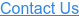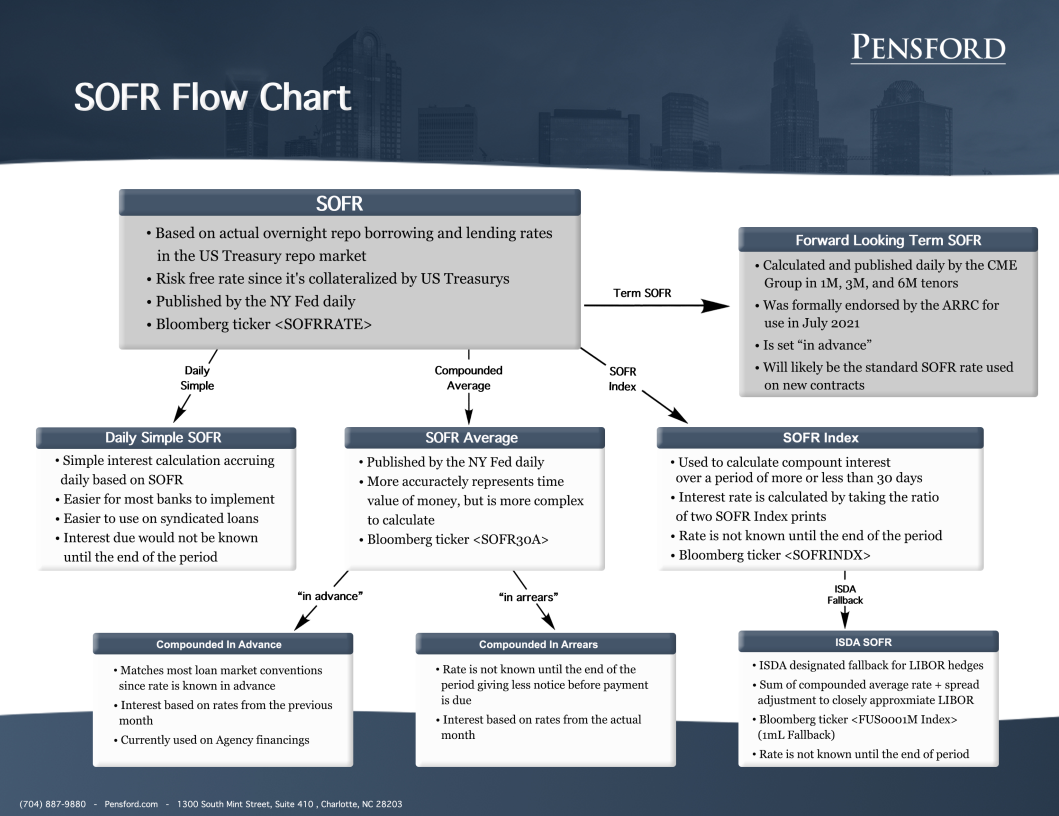# SOFR Rates For Dummies - A Helpful Overview in Layman's TermsOverview

There are various SOFR rates/calculation methodologies which often leads to confusion.  In this resource we touch on the key differences between the various indices to help shed some light on what is what.

SOFR

This is the volume weighted median derived from the borrowing rates of actual repo transactions, or the cost of borrowing cash overnight collateralized by Treasury securities.  For that reason, it’s often referred to as overnight SOFR.

The NY Fed calculates and publishes SOFR each morning using the data from the preceding business day and it’s the basis for all other SOFR calculation methodologies.  You can find the updated data here or on Bloomberg under ticker <SOFRRATE>.

Daily Simple SOFR

This is a simple interest calculation.  The hedge/loan accrues interest daily based on SOFR and the total amount due is known at the end of the period.

If the notional is constant, the total interest accrued would be the same as applying the simple average of the SOFR resets at the end of the period.

SOFR Average

This is the compounded average of SOFR from the previous 30, 90, or 180 days.  In other words, it’s a rolling compounded average which is updated daily by the NY Fed after SOFR is published.  The NY Fed publishes SOFR Averages here and 30D SOFR can be found on Bloomberg under ticker <SOFR30A>.

Since SOFR Average is compounded based on the preceding SOFR prints for the respective term, it’s often referred to as compounded SOFR set in advance and is the index currently being used on Agency financings.

Loans based on SOFR Average are technically using SOFR from the previous month, but since the rate is set in advance like LIBOR, borrowers still know their rate at the beginning of the period.

Compounded In Arrears

SOFR Average can also be used “in arrears”.  This means that the interest due is based on rates published during the interest period, but the payment would not be known until the end of the period.

Whether you’re using a compounded average set-in advance or set in arrears, interest rate hedges are available from several counterparties.  Read more about Agency SOFR caps here

SOFR Index

SOFR Index is used to calculate the compounded average of SOFR over a custom time period.  In other words, it can be used to calculate the compounded average of SOFR over a 28 or 31 day period, whereas SOFR Average would always be 30 days.  The NY Fed publishes SOFR Index alongside SOFR Averages here and the index can be found on Bloomberg under ticker <SOFRINDX>.

The sole value of SOFR Index on any given day is of little value, but this is because the index is a means rather than an end.  To calculate the interest rate, the ratio of two values (the period start and period end) are used to calculate compounded averages.

SOFR Index is based on the same calculation methodology as SOFR Average, so calculating the rate using the same start and end dates as SOFR Average should produce materially similar results.  Note, there will likely be minor differences due to rounding.  Here’s an example comparing SOFR Index and SOFR Average:

Date                          SOFR Index

3/2/2020                     1.04085026%

4/1/2020                     1.04136820%

[(1.04136820% / 1.04085026%) – 1] * (360/30) = 0.59713% which matches the 4/1/2020 30D SOFR Average print.

ISDA SOFR

This index is calculated and published by Bloomberg under ticker <VUS0001M Index> and is being used as ISDA’s replacement for LIBOR based hedges.  This variation is also a form of compounded SOFR in arrears and is the sum of (i) the Adjusted Reference Rate and (ii) the Spread Adjustment, each for the relevant LIBOR tenor.

• This is a compounded average of SOFR over the accrual period. Since the rate is calculated in arrears, the reset for that accrual period won't be known until approximately 1 month after the reset date for the period in question.
• Said another way, if the LIBOR rate for the August period was based on the 7/28/2022 LIBOR reset, then under ISDA SOFR the rate would not be available until 8/28/2022.

• Since LIBOR is an unsecured lending rate and SOFR is a secured overnight rate, LIBOR has historically been higher than SOFR, so a spread adjustment is necessary to make SOFR more comparable.
• The adjustments, which were fixed in March 2021, were based on the five-year median difference between LIBOR and SOFR.

Since ISDA SOFR is meant to serve as a replacement for LIBOR, the difference between the two rates is minimal.  Check out our white paper here for more on how the transition will change your rate.

As outlined above, ISDA SOFR is set in arrears where LIBOR was set in advance. In lieu of the rate being determined two business days prior to the beginning of the interest period, it will be known two business days prior to the end of the period.

Forward-Looking Term SOFR

The creation of a forward-looking term SOFR index based on derivatives markets was the final step in the ARRC’s Paced Transition Plan. This index is desirable since it would be set in advance like LIBOR, so the payment is known at the beginning of the period, and is credit sensitive which eliminates the need for an additional spread adjustment.

On July 29, 2021, the ARRC formally recommended the CME’s forward-looking term SOFR rate for use, checking the last box of the Paced Transition Plan. Banks have since started issuing debt and selling hedges based on Term SOFR. This index can be found on Bloomberg under ticker <TSFR1M>.

Conclusion

With multiple SOFR indices available for use, in addition to rates such as Bloomberg’s BSBY (read more about BSBY here), borrowers may find themselves with a term sheet listing an index they’ve unfamiliar with.

One interesting fact we came across is that the difference between a simple and compounded average of SOFR over the previous two decades would have only ranged from 0-10 bps. Given how similar the terminology and rates are, it can be tricky to determine which index is being discussed, but at the end of the day they all shake out to be about the same. When in doubt, please reach out and we’ll be happy to assist.

Below we’ve included some helpful links if you’d like to dig in more. Should you have any questions, please let us know.